Select PageWe left off Chapter 32 Part W, with a nod to the parameters of Chaos Theory being embedded in the encasing pyramid, a preserved remnant from a previous 12,000-year cycle. Now we would like to go a bit further back in time, back before physical existence.  The last 3 articles tell a story unto themselves that recaps key areas of this on-going book and swiftly moves us into the future, though The Journey into the understanding of Spherical Time began in earnest with the start of Chapter 32.

As previously explained, the Hebrew Letters are the necessary interface between the cosmic letters, the concepts behind the 10 digits 09, and with physicality.  This is not to say that the digits should be idolized or that they are exalted symbols; they are representations of conceptual forces, like the 10 sefirot beyond our true comprehension. They themselves are also interfacial, albeit at a higher dimensionality. The Hebrew letters transcend physicality, at once linking to it and guiding it and but also embracing all that is spiritual and cosmic in nature.  They are what we call nature.

There are 27 of them when the 5 final forms are counted, and they go by 22 Names.  Originally there were only 10 of them (דוזינכסרךן), one for each cosmic digit and sefira, but they merged into 50 component letters to form 27 independent shapes or archetypes that gave rise to physicality.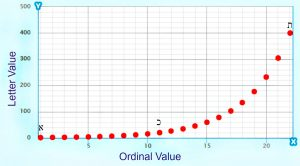The cosmos or hyper-dimensions transferred a numerical value based on an exponential scale to each letter/archetype. It is simply 1.313x whereby x is the ordinal value of the letter within the Hebrew alphabet and 1313 is the year 1313 BCE that the Torah was given to the Israelites at Mt Sinai of numerical value 130. Both these numbers have primal cosmic significance as 13 is both the numerical (13) and ordinal value (13) of Echad (אחד), One, as in “G-d is One.” The numerical value of G-d, YHVH (יהוה) is 26, as in the complete value (13 + 13) of Echad (אחד), One. Oneness, unity, is a concept, a construct, a primal one within the conscious network of the universe. So, the combination of the two cosmic letters that form the word 13, while just a number to us, are profoundly significant to the structure of the universe.  Picture them not as numbers but as the most powerful force of unconditional love that you can imagine.  Start with the love you may feel for your children, parents, or pets and imagine it building and increasing exponentially from there.  These are forces, not numbers, and the symbols that represent them were carefully chosen by the Creator long before he chosen to make Man.

As each of the 27 letters’ value is based on the ordinal value of the letter within the Alef-bet, we must assume that that position is of the utmost significance. More so than the letter, the symbol, itself. In spirituality, what comes first is always the most important; everything else follows from there. Because the physical world is the world of illusion, no one really knows what came first. History is rewritten daily.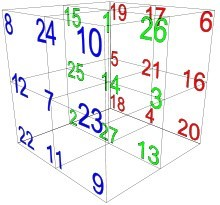We know that the 27 ordinal values are laid out according to a specific 33 matrix cube in 9 sets of Triplets, (1-2-3, 4-5-6…25-26-27) along alternating planes and axes, and that their positions were designed so that that in every direction along every plane, axis, and every centered diagonal the triplets would sum to the singularity, 42. Like 13, 42 is one of the most primal concepts in existence. From their fusion (13 x 42) came the seeds of the 10 sefirot of the Tree-of-Life. The cosmos communicates that to us in that (13 x 42) = 546, the sum of the 10 initials of the 10 sefirot (dimensions), and from these came the 10 digits and the 10 original Hebrew letters, but none of this is comprehensible if you see 13 and 42 as passive numbers and not active living concepts. Perhaps if you recall that numbers are only counting tools and envision them as 13 somethings and 42 somethings that react together in specific ways within the mind of the Creator.

When we say that the numerical values associated with each of the 22 letters are on an exponential scale, we mean that they increase in value exponentially from letter to letter. In other words, they fit an exponential curve, such that y = abx where y is our letter value (for example Alef (א) = and x is the ordinal value of any of the 22 letters counted from 1 22 (for example Alef (א) is 1, as the first letter), and b is the factor that guides the exponential rate of growth.  The value b is a constant, unchanging as x rises. This does not mean that every letter value falls directly on the curve, only that the curve (exponential model) closely fits the data (the letter values), and that the starting value (1) and ending value 400 for the letter Tav (ת) are directly on the curve and in this case, the 11th letter Caf (כ) of value (20) is bang on as well.  Astonishingly, in the case of the alef-bet, that key factor (b) is 1.313.The 3 Hebrew letters Alef, Caf and Tav anchor the curve at its ends and midpoint, while the other letters are slighting above or below the curve.  These 3 letters match 3 of the 4 nucleotides in out DNA, with the 4th one having the value 3, thus their total value is 424, that of Moshiach Ben David.The 3 Hebrew letters Alef, Caf and Tav anchor the curve at its ends and midpoint, while the other letters are slighting above or below the curve.  This is because they are representations of energy, zones on a spectrum, not fixed points, which believe it or not in mathematics is a very difficult concept. Think of the color green, there are an infinite number of wavelengths for it on the visible light spectrum, all falling within a zone of about 495 nm – 570 nm where the color yellow takes over. The Hebrew letters are the same; they represent a narrow collective or bundle of similar energy frequencies, with each letter at least as different from green is to yellow.

There is yet another reason that we can state that the values associated with the Alef-bet are not arbitrary. Unlike English, these 22 Hebrew letters form the initials to their 22 Names and they are concentrated forms of them. For example, the letter Alef (א) of numerical value 1 is spelled out (אלפ), whose numerical value when we sum all 3 letters is 111, which is likewise symbolic for the 1st letter of the Alef-bet.

While the example above is quite illustrative, there is a slightly different gematria cipher that extends that exponential scale 5 more places from 22 to 27 letters because 5 of the letters (ךםןףץ) take on a different shape when found at the end of a word, and thus the scale extends beyond 1400 to 500600700800900.  When we sum up the 22 irrational square roots of the spelled-out 22 Names of the 22 letters, we get exactly 424.242…, representing the singularity 42 and Moshiach Ben David, 424, which is an offshoot concept/construct from it.

### The Letters and the Torah

The square root of 6 is 2.449, matching the year 1313 BCE given by the exponent (1.313) that gave us the Alef-bet scale. The log of 600,000, as in the 600,000 Israelite men at Mt Sinai and the 600,000 component letters in the Torah, is 5.778, as in the Event Horizon 5778 HC repeated 3 times in the first 1000 digits in Pi (π), along with its Gregorian analogue, 2019 CE. There are (600,000408) component letters in the Torah and if those 408 letters were Vavs (ו) of numerical value 6, as in the initial of 60% of the verses in the Torah, and as in the 6 bundled dimensions within Zeir Anpin that project onto the 7th and that correspond to the 6 directions of the Essential Cube of Creation, then those 408 component letters would equal 2448, the year of the exile from Egypt and the reception of the Torah, 66.6 jubilee years before 5778.  Again, 6, is a concept and the mathematics of the universe are the relationships between the concepts.  Perfect in every way.

We know that there are exactly 304,805 letters in the Torah and that there always has been. We were specifically commanded not to change a single one of them. Of those 304,805 letters, the 5 sofit (final letters) occur 20,107 times in the Torah, representing the 10 Commandments located in the Book of Shemot Chapter 20 at letter 107,007 in the Torah. Being specifically the final letters, they also reflect the 20 centuries until the 107th Triangular Number, 5778 HC.  The letter Vav (ו) occurs exactly 30,513 times in the Torah, 10.0% of all the letters. More specifically, 30,513/304,805 letters = .100107.

If we take the exact exponential values of the 22 Hebrew Letters before they are mathematically and cosmically fitted to the perfect exponential curve, they form yet another cosmic cipher, one at the level of Binah. Using that cipher, the total exponential value for the 42-Letter Name Matrix is 4262.011, expressing the singular concept of 42, and 620, keter, the crowning sefira. Nevertheless, when we subtract the 42-Letter Name’s standard value, 3701, we get (4262.0113701) = 561.0107, which not only is similar to 100107 and 20107, but 561 is the sum of the cumulative integer fields from 1 – 33 that are the complement to the cumulative counterspace (34100) = 4489, which is the sum of the 22 exponential value Names, the 22 Names in Binah before they are transformed into an interfacial language that helps bring about physicality, or at least the illusion of it.

The ordinal value of the 42-letter Name is 524, and (561.0107524) = 37.0107, the original Zeir Anpin value of the 42-Letter Name Matrix, 3701, coupled with its palindrome, 107, or alternatively, the 7 lines/levels of the Matrix that correspond to Zeir Anpin/Malchut.

The 11th and midpoint letter Caf(כ), the linchpin between the Binah exponential scale and the interface transitioned scale, of numerical value 20 on both scales is found 8610 times in the Torah, representing 2.8248% of all the letters, yet another Torah connection to the ubiquitous 248 dimensions, and to 28, representing Koach, power.

The letter Alef (א) on the other hand, is found 27059 times and represents 8.8775% of the Torah, as in 5778 backwards.  The letter Tav (ת) on the other end of the spectrum is found 17950 times, giving it 5.889% of the total letters in the Torah, or 5.778 plus .111, as the Name of the letter Alef (א). When they are added together, they represent 14.7….% of the Torah as in Jacob’s age (147), and when Caf(כ) is then added, together they equal 17.5…% of the Torah, as in Abraham’s age (175). Then finally when the 4th of the 4 letters that represent the 4 nucleotides in our DNA, the letter Gimmel (ג) is added, together they represent 18.2832%, as in the Jacob’s Name (182), and as in the 832 years that the First and Second Holy Temples stood, and as in the 832 years from the flood in 1656 to the death of Moses and the Israelites entering Israel in 2488 at the end of the 26 generations, 112 years before 2600.

That the 4 letters have a nominal value of 424 and that their total percent of the Torah is 18%, whose square root is 4.24 is cosmically telling enough, but that the square root of their exact 18.2832% is 4.275, as in the Primal Frequency 27.5 Hz explains a lot more. This must all be balanced out with the 90,100 Yuds(י), Heis(ה), and Vavs (ו) in the Torah, as in 90100 is the 424th Triangular Number. Remember the commandment to not change a single letter. There is a reason the Torah needed to be preserved perfectly intact for us today, in spite of Man’s desires to rewrite all that came before him.

Whenever Man rewrites history he is attempting to change the simulation in which you live.  He changes the systems and the laws, makes up the physics and dimensions of the universe, the ethics and morals upon which you are allowed to base your decisions.  As he shifts civilization’s moral compass, so does the Earth.  The actual simulation and rules that govern it do not change, but what does that matter to you if you cannot see it. If all you see is the illusion then that becomes your life, your prison, rather than the path to the ultimate freedom of expanded consciousness which it was originally meant to be. As the Earth tips, so does the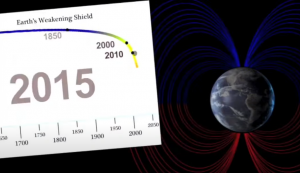sands of Spherical Time. Right now, the magnetic poles are over Siberia and the South Atlantic, not Santa’s wonderland and Antarctica. Over the past two decades they have been accelerating toward the equator at 5% a decade and will soon be at 5% a year. There will be a tipping point, a point of no return. We are already 5 years into the Event Horizon, the stretching zone where the torus of Spherical Time folds back upon itself and the pathways of time intermingle and become confused. You can call it the Mandela Effect.  Until the tipping point and the real great reset occurs there is still time to focus the processing power of your mind and link up with your consciousness. Think of these darkening days as the days of mercy, our opportunity.

### Exponents

While the Hebrew alphabet is wholly derived from an exponential scale based on a factor of 1.313, this is the inverse of the logarithmic scale, and the logarithm of 13 is 1.11, mimicking the numerical value (111) of the first letter, Alef(אלף).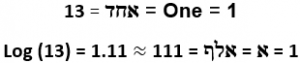We know that it is astonishing on its own that the total words, letters, and verses in the Torah total 390,625 or 58, but now we can better understand why the 5th root of 390,625 is 13.132639022019…, circling back to the 1.313 of the Alef-Bet; the YHVH (26); the 5th word of the Torah (The Heavens) whose value is 5390; the 22 Hebrew letters; and 2019, the last of the 1000 digits in Pi (π) and Western analogue to 5778 HC and the commencement of the Event Horizon.

The 12th letter Lamed (ל) of complete value 42 is special for another reason. On the exponential scale, the letter Lamed (ל) = 1.31312 = 26.2528…  If we flip the exponential power with the variable ordinal value of the Alef-bet the letter Lamed (ל)’s spectral value becomes 121.313 = 26.1195…. This entire second spectral scale ranges from 1 to 57.889 from Alef (א) to Tav (ת), and the only letter that has a very similar value in both scales is the letter Lamed (ל). And while 26 is telling on its own as the value of the YHVH (יהוה), the average between the two scales is (26.2528 + 26.1195)/2 = 26.18618625 or Phi(φ)2, 2.618; Phi(φ), 618; and 625, as the square root of 390,625, the total of the 3 basic Torah elements.

Another important attribute of the x1.313 scale is that the total for the 22 letters, 57.889 is (55 + 2.889), as in the important double cycle (55) of the Primal Frequency (27.5 Hz) and the midpoint in the 5778 radius, 2889 HC, of Spherical Time.

A 3rd significant cosmic concept is symbolized by the number 55. It represents the double cycle of the Primal Frequency (27.5 Hz). It sequentially is represented in the Torah, mathematics, and the universe as 13, 42, 55, which are the 3 central figures in the 7 exceedingly rare Bell Prime Indices, as we have previously analyzed, and in the 13 Vav (ו) initials in the first 42-word paragraph of the Shema, and in the 42 Vav (ו) initials in the rest of the Shema, and the 55 Vav (ו) initials in the entire 248 word, 1000 letter Shema.

### Deviations from the Curve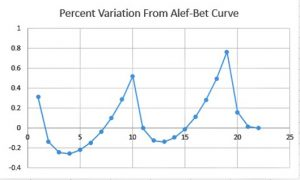This is a line graph without the curves being smoothed out, but you can already notice a nice curved repetitive pattern, growing fractally as the alef-bet progresses. Notice how that curve peaks at three points, at the Alef(א), the Yud(י), and the Koof(ק) of numerical values 1, 10, and 100 respectively in exponential progression, or geometrically by a multiple of 10. They total 111, as in Alef (אלף). While their ordinal values progress arithmetically by 9: 1, 10, 19. The value of the 28th letter would be 1000.

One further side note is that the ratio that the letter Koof (ק) is off exactly and percentagewise from the 1.313 exponential curve compared to how much the letter Yud(י) is off is 244.8, as in the year 2448 HC (-1313 CE) that the Torah was received at Mt Sinai.

When we extend the graph to what would be the 28th letter at value 1000, we get the same extended fractal image of the percentage variations from the curve.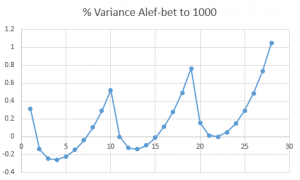And the slope between the fractally corresponding points is 1.6.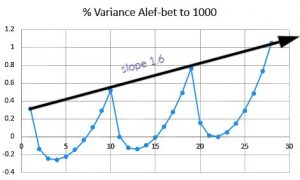What is most interesting is that that slope line is also the Alef (אלף) line, since the values 1, 10, and 100 and their corresponding letters Alef(א), the Yud(י) and the Koof(ק) are on it.

As we can see in the next graph, where we flipped the variance curve along the Alef slope axis, is that the curves are indeed symmetrical.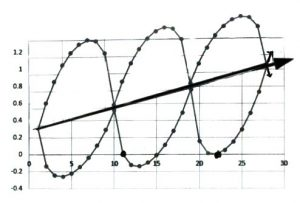We can also see that it is a sine wave or at least based on one. It is the bottom half of an out of phase sine wave where the two waves cancel each other out.  It also conforms to the top half of a longitudinal wave.Now, remember, the actual Alef-bet curve looks like this, not a straight-line axis, so this sine wave is actually symmetrical along the exponential curve.This should remove any doubts that the Alef-bet was meant to fit this curve based on 1.313 and the concept of One.  So why this symmetry to the Alef (אלף) axis, whose slope is 1.6 or approximately Phi(φ)?

When we add the absolute value for all 27 percentage variances, they equal 6.1823 or approximately 1/Phi(φ) or .618033….  Moreover, the 21st letter, Shin (ש) has a variance off its nominative value (300) of 4.48033… or 1/Phi(φ) – 1.70000…. And since 17φ = 27.5, the value of the cubit and the Primal Frequency: 10varשφ= 27.5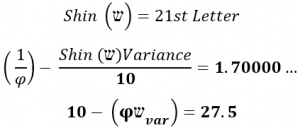The concept associated with the letter Shin (ש) and 21 must have profound significance that incorporates this equation because the sum of the first 21st Names of the Letters equals 3842, the sum of the first 42 letters of the Torah. And they all connect to the higher Name of Binah, Ehyeh (אהיה) of numerical value 21, the Name to which the 42 Letter Name Matrix is our channel and ladder.

The Alef-bet begins with the letter Alef (אלף) of numerical value One (1), but its deviation from the curve is .313, making its exponential value 1.313 as the starting point for both the Hebrew alphabet and the Israelites at Sinai (1313 BCE). This is also the starting point of their 42 Journeys and of their counting of Jubilee years (50-year increments), almost as if time began at that moment and everything before it was an implanted confabulation.

We explained that the Hebrew letters in their pure form represent a vibrational range and not specific values. Now we understand that the range for each letter is a percentage variation from the curve conforming to a specific wave function with a specific slope.

That vibrational range equals the amplitude. The formula tells us Amplitude = how much Energy is being transmitted by the wave.As the full wave cycle intersects the Alef (אלף) axis at Alef (א) and Koof (ק) of ordinal values 1 and 19, the wavelength is (19 – 1) = 18, as in the square root of 18 being 4.24264068… a representational conglomeration both 42-Letter Names, Moshiach Ben David(424), the YHVH (26), etc., and a networked link to Phi18 = 5778.000

### 22 Letters and 27 Names

Each of the 27 letters has a specific value of 1.313x regardless of what percent variation it is on or off the curve.  Each of those same 27 letters has a letter value related to that curve, and a spelled-out Name, which is cosmically one dimensionality higher.

There is no physical relationship between these numbers. For example, Alef (א) is 1 and Alef (אלף) has a sofit value of 831. If we take the sofit Name value over the simple letter value, we get 27 different ratios, ranging from 831/1 or 831 to .10125.

The sum of those 27 ratios is 1497.626845, and if we subtract the total letter value (1495) for the 22 regular letters out of the 27, we get (1497.626845 – 1495) = 2.626845, which equals (2 x 1.3134226) or twice the exponent value (1.313) to which the entire Alef-bet is based upon.

#### (The sum of 1- 27 Names)/(the sum of 1 – 27 Letters) – (the sum of 1 – 22 letters) = 2 x the Exponential Coefficient

What makes this extraordinary equation even more mind-blowing is that (1.3134226 1.313) = .0004226 and 4226 = the sum of the 22 Names less the kolel (22): (424822) = 4226.

#### (The sum of 1- 27 Names)/(the sum of 1 – 27 Letters) – (the sum of 1 – 22 letters) = 2 x (the Exponential Coefficient + (the sum of the 1 – 22 Names – 22)/107

While it might seem like these values might somehow be mathematically related, there is no physical reason that they should be. This multi-dimensional systemic organization illustrates a definitive design to firmly tie the Alef-bet and their 22 Names to their 27 spatial positions while anchoring them to the Path and Network of One.  They are cosmically bound.

What we do know is that the there must be a connection between this deliberate resultant 1.3134226, the Primal Frequency 27.5 Hz, and the 7 dimensions of Zeir Anpin that determine physicality because 27.51.3134226 = 77.70

For what it is worth 1/10th of the 5th root of 390,625 or 13.132639022019… is off from 1.313422619 by 1/6300.

### 27 Positions; 26 Spokes

Since the valuations of the entire Alef-bet is based on 1.313 raised to the 27 ordinal values it is obvious that the 27 ordinal values are what came first.There are 27 positions in the Alef-bet corresponding to the 27 positions in the Essential Cube of Creation. The central position in that cube and alphabet is the letter Nun (נ) of numerical value 50 and ordinal position 14. It is one of the original 10 component letters, and there is 14,126 letters Nun (נ) in the Torah, as in its ordinal position (14) within the Essential Cube of Creation and the 126-value of every face, plane, and the 3 axes that make up that Essential Cube of Creation.  One could even point out that the sum of digits in 14,126 equals 14.

It represents or contains all 50 component letters that the original 10 will spread into to create the Torah. This one of the reasons the Gates of Holy Temple are 10 cubits wide and 50 cubits high.

When visualized within the Essential Cube of Creation we see that there are 26 spikes radiating off the singular central position, leading to the rest of the letters, begging the question, “What came first the 26 spikes or the 26-value of the YHVH (יהוה)?”

The spikes are both vectors and pathways, 26 of them, and their distance is measured in the differences in their original values.

That distance is measured by the square of those differences and by the cube of those differences or of the absolute value of those differences if they are calculated negatively. Since the difference in the letter Nun (נ) or (1414) = 0, that vector will always be “0,” so the results are the same for all 27 letters as they are for all 26 vectors/pathways.  The sum of the 26 or 27 squares is 1638 and 16562 for the sum of cubes. The total distance for the sum of the 26 squares and cubes for the 27 letters is (1638 + 16562) = 18200, as in the 1820 YHVH (יהוה) in the Torah, each of numerical value 26.

Those 1820 YHVH (יהוה) and the 18200 sum of the 26 Essential vector distances are directly connected to the Path of One, the sequential summation of the series of order of 13, that begins with Echad (אחד), One: 113914551820.

There is another primal cosmic connection to 18200 that runs through the ether and gives us a deeper understanding into the nature of universal consciousness.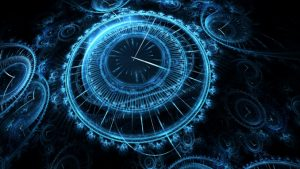As explained in depth in Chapter 28 Spherical Time – Frequency, there are two unique Primal Frequencies based on modulus mathematics and the multiple of 9, Tesla’s secret codes. These two Primal Frequencies 27.5 Hz and 1.1 Hz run concurrently and phase together at specific intervals, which we will get to momentarily. Meanwhile, they have special properties that connect to the Phi(φ) angle (137.5o) at the core of Spherical Time, to the YHVH (יהוה) value, 26, and to the Cosmic Harmonic 1.273, or 4/π.

We have previously explained that the 9 digits or 10 with “0” are the cosmic letters of the universe and everything else is composed of the words they form and the grammar or relationships between them. The simplest grammatical component is the ratio between the digits. There are 81 or 92 basic interactions or possible ratios between the 9 digits, matching the (79,976 + 1024) = 81,000 words and word values in the Torah.  Those 81 or 34 ratios total 127.30, giving us new and more basic understanding about the Cosmic Harmonic, 1.273, or 4/π.

Moreover, the sum of the 81 possible additions between the 9 digits is 810 and the sum of the 81 products is 452 or 2025, making their 81 P/S ratios equal to 173.800, as in the gematria katan (173) of the 42-Letter Name, the 173 Keys to Heaven given to Moses.

There are 26 generations from Adam to Moses, like the 26 vector spokes, and the net total difference from generation to generation is 810. Another way to understand this Adam lived 930 years and Moses lived 120 thus (930120) = 810.

The total of the absolute values of those net differences from generation to generation is 2702, or 1 more than the value of the Torah’s first verse, or alternatively One (1) over the value of the 42-Letter Name (1/3701).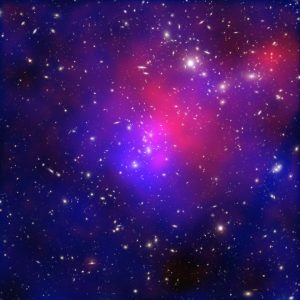Because of the way the cosmic system is designed, including the Alef-bet interface, the 27th Letter, Zadi Sofit (ץ), has a squared (902) value of 8100, and a cubed (903) of 729,000, matching the 2nd tier (729 or 93) of the 42-Letter Name. Its Pi(π) Phi(φ) gematria value is 273, as in the cosmic harmonic and the ultimate temperate, Absolute Zero, 273 K or -273o C.. This compares with the complete 2Pi(π)Phi(φ) gematria of all 27 letters, 5778, as in the surface temperature of the Sun, 5778 K and 2889, as in the surface temperature (288.9 K) of the Earth, the (Genesis (רץ) + Phi (φ)) cipher value for those same 27 letters.

Simply, the two Primal Frequencies together (1.1 + .275) = 1.375 or (27.5 + 110) = 137.5, the Phi(φ) angle (137.5o) that turns our universe within the circle.  As previously noted, the Phi(φ) angle (137.5o) corresponds to the 50-cubit height of the 11 Gates of the Holy Temple, 1375” as in the central letter Nun (נ) that resembles those tall gates.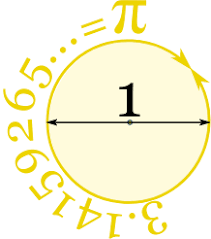The circle is created by the primordial ratio. The two frequencies combine to power the circle to turn at just the right amount to etch Phi(φ) upon it: (1.1 + .275) = 137.5o. They can also combine, like the 81 ratios of the 9 digits, to close it off, inscribe it, as (1/27.5 + 1/11) = 1.273 or 4/π.

This all works because the frequency 1.1 is exactly 4% of 27.5.

The two frequencies that resonate with the number 9, run side by side, 27.5 Hz and 1.1 Hz. When you hear “frequency” you should picture sine waves, and a specific number of crests within a single unit of measure. In this case 27.5 and 1.1, with the 1.1 wave 25 times longer (from crest to crest) than the 27.5 one. The 2 of them will be naturally out of sync but line up or crest at the exact same moment periodically. It works out to 2 pulses of 27.5 for every 50 of 1.1.  Both at the value and subsequent multiple of 55, as in the primal cosmic sequence 134255. We must assume at this point that this has something to do with the numerical value (50) of the central letter Nun (נ), whose first vector stretches to the number 1 and whose last stretches in the exact opposite direction to number 27.

Mathematically, that works out to (2 x 27.5) = (50 x 1.1) = 55, as in the height of the entrance to the encasing pyramid, etc., etc., At this point, the wave height is at its maximum, twice its normal crest. It is also designed so that 55 is the sum of the numbers 1 to 10.

The two scales of Primal Frequencies run in cycles of 11 with 10 numbers that have endless remainders in cyclical multiple of .0909… in between them. Every 11th or crowning result gives us a whole number or a whole number when multiplied by 10. The 11th result is equivalent to the sefira of Keter, and the 10 in between to the 10 sefirot of the Tree-of-Life including Da’at.

The cycle for 27.5 Hz always begins (.363636…,.727272…,.909090….), corresponding to the 3 highest sefirot and equaling 1.9999999, or 2. They are sequentially always followed by (.454545…,.818181….181818…,.545454…,.090909…,.2727272…, and .636363…,), which correspond to the 7 lower sefirot of Zeir Anpin, and which summarily equal .29999999 or 3.  The 3 upper sefirot thus equal 2/5 or 40% of the cycle and the 7 bundled lower sefirot equal 3/5 or 60% of the cyclical remainders.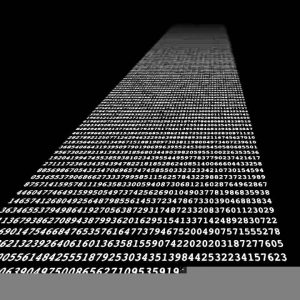Like all the other keys to the cosmos, their total of 4.999999 is represented in the first 1000 digits in Pi: …4999999… is found at digit #762, which is 238 or 1/42 from 1000. In terms of gematria 238 is the matriarch, Rachel, and mathematically, the value 7.62 is the sum of the 10 cube roots of the 10 cyclical remainders. Nothing is ever out of place in the design of the cosmos. The chaos we see in our physical projection of the universe is just an illusion. Once you are truly tapped into the consciousness from above that all vanishes.

That the 10 remainders total to 5 is of utmost significance and places them at the core of Phi(φ) based on the square root of 5, and of the Torah, whose 3 main elements total 58.

It also means that the 10 resultant values for the 10 first integers, 110 add up to exactly 50, as in the central letter Nun (נ) at the core of the Essential Cube of Creation and as in the core of Spherical Time with the Phi(φ) angle (137.5o) or (50 x 27.5 Hz). The product of the 10 remainders is .0001399 or 14, also at the core of both the Essential Cube of Creation and Spherical Time.

We have often said that the Torah is based on or at least integrated with the 248 dimensions of the E8 Lattice, and we have shown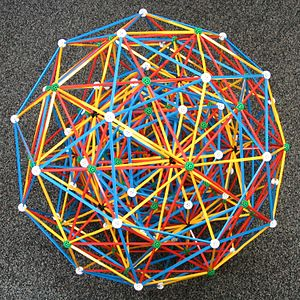its myriad connections to 248. The E8 Lattice of the E8 Lie Group—a special, even exceptional type of mathematical symmetry—has a rank 8 root system containing 240 irreducible root vectors spanning R8 and all of the same length.  Of those 240 root vectors, 112 have all 8 of its coordinates that are all integers. The rest, 128 or 27, have all 8 as half-integers. All the coordinate groups that comprise the root vectors have even sums.  Those 112 roots with integer entries form a D8 root system, and yet another subset of the E8 root system contains a copy of A8, which has 72 roots.

While the concepts, as referenced by the numbers, are symmetrical and constant throughout the consciousness that is the cosmos and are made simple so that we can connect to them, there is no underestimating or under-appreciating their enormous complexity.  One (1) over the sum of the 8th roots of the 10 cyclical remainders is .111321…, and it is precisely 1.016992 less than 10, with 16992 being the sum of the 5 Essential Elements of Creation (the 4 sets of Triplets that total 112 Triplets and the 27 positions of the Essential Cube of Creation), which are the 5 elements that comprise the 363 letters of the Cosmic Wheel, as detailed throughout Chapter 32.

We should also point out that the sum of .090, .181, .272, .363, .454, .545, .636, .727, .818, and .909 equals 4.995, as in the sum of the 27 letters of the Alef-bet.

These are the same 10 cyclical remainders for the 1.1 cycle, except those are in a different sequential order and split almost down the middle totaling 2.4545 for the first 5, and 2.5454 for the second 5.

Both Primal Frequencies beat every 11 integers and sync up every 5 groups of those beats. As we divide the set of all integers by 1.1, the results get incrementally closer to a whole number every 11th integer, this is one beat. Those whole numbers are in increments of 10. As we similarly divide by 27.5, the results get incrementally closer to a whole number every 55th integer; this is one pulse. Those whole numbers are in increments of 2. Every 11 integers the results get incrementally closer to a whole number when multiplied by 10; this is one beat. Those whole numbers are in increments of .4, so when multiplied by 10 it gives us 40.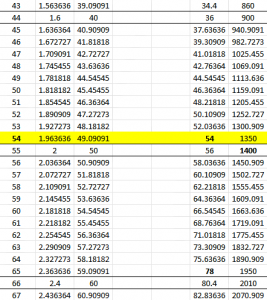Therefore, while the ether beats every 11 integers for both frequencies or scales, 5 simultaneous beats each in every 55, or 10 between the 2 scales, there is only one pulse of the 27.5 Primal Frequency for every 55 integers.

Why is this important? Because something must connect the myriad digital networks throughout the universal consciousness, and we know that something cannot be physical, as physicality is lower dimensional. As below so above. We have beating hearts, so does the cosmos and the universe.

These two separate yet parallel pathways form bridges to one another, besides the one every 11th rung or subtle beat, and they have certain equilibrium points that are quite extraordinary. These are the phase points, like 55. On the other hand, the inflection point is when the cumulative n-mod 27.5 frequencies gets closer and closer to the matching nth integer dividend at number 54, as in the 54 cycles of the Torah and of 107 years that total 5778, the Spherical Time radius and Event Horizon. Beyond 54, the numbers get further and further away.

### Phase Points and the 26 Patriarchs

While 55 is the first of the known phase points of the two scales/cycles, the cumulative sum through the 55th integer of the 1.1 scale is exactly 1400, as in position 14 at the center of the Essential Cube of Creation. Moreover, 55/1.1 = 50, the letter value corresponding to that 14th position, the letter Nun (נ).

At the 10th pulse of those 11 beats, corresponding to integer 110, the cumulative total for the 27.5 scale is 222 and it is 5550 for the 1.1 scale, or 5772 cumulatively for both. This is the 2nd phase point. We know from Rav Ashlag, the significance of 222 = (60005778), so (222/1.1) being equal to 201.8, as in 2018 CE (5778 HC) is probably yet another cosmic analogue to the Event Horizon date.  Meanwhile, the value 5772 is also Euler’s Constant (.5772156…) and 156 is the numerical value of Joseph, who lived 110 years.

And speaking of Joseph (156), the sum of the values of both scales together for the 165th integer, as in the midpoint score lines (165 cu) in the bases of the encasing pyramid, is 156. This leads to the sum of the cumulative values of both scales through 330, as in the encasing pyramid’s base’s 330 sacred cubits, being exactly 312, or (2 x 156), the measure of the Outer Courtyard of the Future Holy Temple.

Abraham lived 175 years and the cumulative sum of the 1.1 scale is 14000 for the 175th integer, again as in position 14 at the center of the same Essential Cube of Creation. Then a very odd things happens when we get to the cumulative sum of all the integers through 275; the 1.1 scale sums to exactly 34500, as in Moses (345), and the integer phase for both scales sums to 260, as in the YHVH (יהוה). Meanwhile, the cumulative sum of both scales totals 35880 as in Moshiach and Moses’ age (80) at Mt Sinai, and the 80 times the numerical value of Moshiach, 358, is utilized in the Torah.

Jacob, the 22nd in the Adamic lineage, lived 147 years, which we saw incorporated into the sum of the first 27 Partition Function Numbers, 14741, so it must be cosmically relevant that the sum of the square roots of the 27 letters of the Essential Cube, as vector pairs centered on the central letter Nun (נ) equal 147.1126, as in the 112 Triplets and the value for the 8 corners of the Cube, and as in the 126-values for the faces, planes, and axes of the Cube. As it must be cosmically connected that 14147 is the 42nd all-inclusive Triangular Number, which is the sum of the 42nd Triangular Number—the cumulative sum of the first 42 integers—with the cumulative sum of the first 42 Triangular Numbers, in other words the 42 of the 42.  The Essential Cube of Creation is firmly anchored in the singularity, 42, the thus the Hebrew Alef-bet, the Torah, and all physicality is as well.

Abraham, Jacob’s Grandfather, was the 20th generation from Adam, and as we know he lived 175 years, so the cosmic synchronous alignment with the 20th all-inclusive Triangular Number, 1750, must be intentional, as is the 20th Triangular Number 210, the height of the encasing pyramid and the Tower of Truth.

The value 210 is part of a Number Theory algorithm or cosmic alignment whereby the resultant value for every 6th integer or integer wholly divisible by 6, will tend toward a whole number. In this case 210/6 = 35, making it the 35th such number. The value 42 is the 7th such number. The nth all-inclusive Triangular Number divided by n2 will tend to the whole number (n/6 + 1). The higher the integer (n) the closer to a whole number the result will be.  In the case of 210 that result is 36.003.

Another cosmic alignment that is important to Spherical Time is the life of King David who lived 70 years and the midpoint in his life, his 35th year, marks the midpoint from Adam to Moshiach, the midpoint in the 5778 Event Horizon and radius, 2889 HC.  Lemehk, Noach’s father, lived 777 years and the 35th sum of the Triangular Numbers is 7770, which matches the Primal Frequency 27.5 Hz, raised to the power of the Alef-bet exponential variable 27.51.3134226 = 77.70.  It all comes together because it must. It is a closed system.

It is not that this is the Alef-bet exponential variable, etc., it is that the Alef-bet and universe was formed around this arrangement of numbers, the cosmic letters that spell out Oneness and Love of the Creator.

It all comes together because it must, because it all has the same singular source and nothing in the universe is random.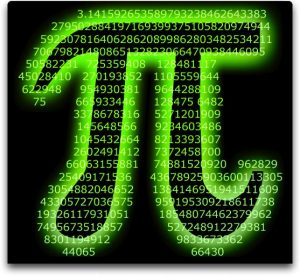Applying that same all-inclusive algorithm to 42 gives us 14741/422 = 8.0198, which is reminiscent of the final 5 digits within the first 1000 of Pi (π) …420198.  Within those 1000 digits there are only two repeated 5-digit or higher numeric strings, …42019956112… at digit # 702, as in the value of Shabbat (702) and 42019 again at digit # 995 …216420198. While the value 956 in the first sequence …42019956112… can be said to represent digit #956 where we find the final of the 3 strings …5778…, the “112” is an overt connection to the 112 Triplets, as is the 216 in …216420198, since the two sets of Triplets, the 216 Letters and the 42 Letters, are integrally related.

Noach was the 10th generation, and the 10th all-inclusive Triangular Number is 275 and the cosmic system is designed such that its algorithm value simplifies as 275/102 = 2.75, showing us the fractal nature of the Primal Frequency.

Why are there (600,000408) component letters in the Torah and not 600,000 which would seem to be more fitting? Everything about the Torah is deliberate, including the year of the Flood in 1656 HC. Those 1656 years times 7 equals 11,592 or (12,00011,592) = 408, and 600,000/12,000 = 50. The Torah is communicating to us specifically about what caused the Flood, the last great reset, and the 12,000-year nova, galactic wave, and magnetic flipping cycles.  Like the 50 component letters in the Alef-bet and the 50 at the center of the Essential Cube of Creation, it all revolves about 50, and the 50th Gate.

The 13th all-inclusive Triangular Number, as in the Path of One, is (91 + 455) = 546, the sum of the initials of the 10 Sefirot (dimensions), and the value of Israel with the kolel. As for the 7th all-inclusive Triangular Number, it is 112, as in the 112 Triplets and the 8 corners of the Essential Cube and as the 112 chakras in our bodies/avatars.

In between the 7th all-inclusive Triangular Number, 112, and the 9th all-inclusive Triangular Number, 210, we have the 8th, 156, the numerical value for Joseph, Jacob’s son.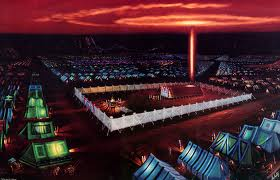The first part of the Path of One is (1 + 13) = 14, the center of the Essential Cube of Creation and of Spherical Time, as the value of David (דוד). Nonetheless, the One over the full Path of One, 1/(1 + 13 + 91 + 455 + 1820) = 42, the Singularity. It is just like the One over the Path of the Israelites, 1/(600000/70/12/3) = 42, and the 42 Journeys they took, traveling with the Mishkan built of 42 elements.

Every cube has 26 elements (8 vertices, 12 edges, and 6 faces) and every centered cube has 26 vectors, yet only one in the universe is based on the Singularity 42. The 26 generations from Adam to Moses came about through the 26 vectors designed into the Essential Cube of Creation, and the total lifespans for those 26 generations is 12600 years, once again referring to the values (126) of the faces, planes, and axes of the Essential Cube. And while the sum of their 26 logarithms of their 26 lifespans is precisely 66.6612, the sum of the 26 square roots is 5358.14805, not only 420 years before the 5778 Event Horizon, but also reflecting Moshiach (358) the central position 14, and the 4805 letters Pe (פ) in the Torah out of the 304805 total letters in the Torah. The letter Pe (פ) has a value of 80, as in the 80 times the value 358 of Moshiach appears as a word amongst those 304805 letters.

Those 12600 years occurred over 2488 years, when Moses passed away and the Israelites entered Egypt, as in the 248 dimensions of the E8 Lattice structure for an average of 5.06, as in the first tier (506) of the 42-Letter Name and the complete value (506) of Moshiach Ben David, the consciousness necessary to access those dimensions.

Arranged as vectors around the central point in the Essential Cube of Creation, the 26 generations would naturally match up with their corresponding positional values and thus there are always two Patriarchs of the 26 counterbalancing the central point, starting with Adam in the 1st position and Moses in the 27th, corresponding to the vertical axis of the Essential Cube (11427). There are thus 13 such paired vectors/pathways, all adding up to 42. The sum of their 13 average values is 6300, as in the difference 1/6300 from what 1/10th of the 5th root of 390,625 or 13.132639022019… is from the Alef-bet variable 1.313422619.

The sum of the 27 positions of the Essential Cube of Creation is 378, the 27th Triangular Number, and the 378th all-inclusive Triangular Number’s square root algorithm is 64.00220, as in the complete value of the central letter of that Cube, 64, showing us why the Creator choose the Letter Nun (נ) for that special hub position. For this to happen, the exact 1.313 exponential variable had to have been chosen as well from the start.

### Deeper Inflections

Another inflection point is found at integer 715, whose 27.5 frequency is exactly 26 and where the sums of the two Primal Frequencies results are 26 and 650 respectively, or 10 times the value of Adonai, giving us 676, which is the also 262. How amazing that so deep into the interaction of the Primal Frequencies the forces of the universe are designed so that the YHVH (יהוה) and Adonai (אדני) would come together in such a unique way.

This is indeed an inflection point because the combined results get closer to an exact square of the 27.5 Primal Frequency result until we reach 26, and then they get further and further away from it afterwards.  It is a singular confluence in the fields, with the 1.1 Primal Frequency being associated with the field and power of Adonai (אדני) and the 27.5 Primal Frequency with that of the YHVH (יהוה), two forces eternally entwined, as we saw with Pi (π) and Phi(φ) within the 42-Letter Name. This time, as they are combined, they release the power of the Light through squaring (262), as Rabbi Chaim Vital of blessed memory advised centuries ago.

We could even say that these are the frequencies of the YHVH (יהוה). This is most significant, perhaps most of all.  The sum of the results for any integer divided by 27.5 and 1.1 then divided by the 27.5 result will always be 26.

We now have a better understanding of how the 26 vectors of the Essential Cube of Creation are connected to the Primal Frequencies of the ether and cosmos.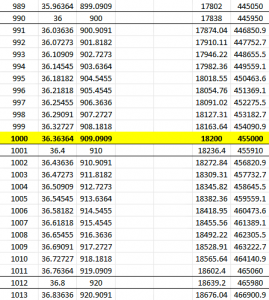Another interesting confluence or phase happens at the 1000th integer, representing Binah. The cumulative value of all the 27.5 Primal Frequency results is exactly 18200, as in Jacob (182) and as in the 1820 YHVH (יהוה) in the Torah. It is even more primally associated with the sum of the 26 squares and 26 cubes of the vectors for the 27 letters within the Essential Cube of Creation, (1638 + 16562) = 18200.

Moreover, as noted, 1820 is part of the Path of One or the order of 13 that beginnings with Echad (אחד), One: 113914551820 and that is built into the Torah and the heart of the super network of the Cosmos. So how apropos that the cumulative value of all the 1.1 Primal Frequency results through that same 1000th integer is exactly 455,000, as in the value (455) of the 3 aspects of Ehyeh (אהיה) times 1000.

If that were not enough of a cosmic synchronicity, the value of that 1000th integer result for the 27.5 Primal Frequency is 36.36363, as in the value of H’Moshiach (363), and the value of that 1000th integer result for the 1.1 Primal Frequency is 909.090, as in the value of Melech, King (90), whose ordinal value is 36, giving it a complete value of (90 + 36) = 126, as in the 126-value of every face, plane, and the 3 axes that make up that Essential Cube of Creation.

Earlier, we saw that the sum of the 27 sofit Names/Letters ratios less the their total letter value (1495) for the 22 regular letters is (1497.6268451495) = 2.626845 = (2 x 1.3134226) or twice the exponent value to which the entire Alef-bet is based upon.

When we apply the same summation of the 22 ratios of the standard Names over the 22 Letters, we get 591.11, which is 906.513 less than the 1497.626845 sum for the 27 sofit letters, and while 906 is the value of the 7th tier of the 42-Letter Name Matrix, 9065.911 is the measure in inches of the West Base of the encasing pyramid, the one related to the chaotic convergent coefficient, and to the chaotic moment.

The encasing pyramid to the Tower of Truth, also known as the Great Pyramid (Joseph’s Pyramid) is a 4-sided pyramid designed around three measuring modalities—the inch, the foot, and the cubit (27.5 inches) based on the Primal Frequency (27.5 Hz—and the relationship or ratios between these measures. There are 4 bases of slightly differing measures and a height of 481.25 feet. Every other measure is derivative of these 5 basic dimensions.

Feet            Inches                            Cubits       Resultant Perimeter inches

Height                  481.25          5775                           210

S Base                  756.25          9075                           330                 36,300

E Base                  755.79826   9069.5791965           329.8029       36,278.3184

W Base                755.4926     9065.9112                  329.6695       36,263.6448

N Base                 755.374       9064.488                    329.6177       36,257.952

Average               755.7287                                                                 36,274.976

Perimeter           3025.00                                                 1440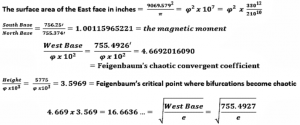The cycle repeats about every 12,000 years, with 6000-year half-cycles, leaving us with ancient remnants of the knowledge built into the cosmos, hints we leave ourselves, breadcrumbs for our consciousness. With the rewriting of history, those breadcrumbs can be gobbled up and Man’s compass lost.  The Torah fills in the history, establishes our moral compass, and hands us the technology, understanding, and keys to the cosmos without having to pretend we are flying into outer space.